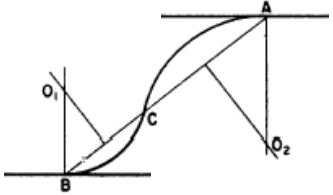Feb 26, 2016

Feb 26, 2016

Feb 26, 2016

# Reverse or Ogee Curve - Definition and Making

## Definition:

Combination of two or more simple circular curves of different radius having their curvature in the same direction. Essentially, a compound curve consists of two curves that are joined at a point of tangency and are located on the same side of a common tangent. Though their radii are in the same direction, they are of different values.

## REVERSE OR OGEE CURVEThis is the process of establishing the centre-line of the curve on the ground by means of pegs at 10-m to 30-m intervals. In order to do this the tangent and intersection points must first be fixed in the ground, in their correct positions.

Consider Figure 8.3. The straights 0I1, I1I2, I2I3, etc., will have been designed on the plan in the first instance. Using railway curves, appropriate curves will now be designed to connect the straights.

The tangent points of these curves will then be fixed, making sure that the tangent lengths are equal, i.e. T1I1 = T2I1 and T3I2 = T4I2. The coordinates of the origin, point 0, and all the intersection points only will now be carefully scaled from the plan. Using these coordinates, the bearings of the straights are computed and, using the tangent lengths on these bearings, the coordinates of the tangent points are also computed. The difference of the bearings of the straights provides the deflection angles (delta) of the curves which, combined with the tangent length, enables computation of the curve radius, through chainage and all setting-out data.

Now the tangent and intersection points are set out from existing control survey stations and the curves ranged between them using the methods detailed below.